# qwraps2: Graphics

There are several graphics generated within qwraps2. The naming convention for the “quick” plots was inspired by the (deprecated) ggplot2 function qplot. The development, flexibility, and robustness of these functions vary. Some “tips and tricks” are provided.

# 1 qacf: Autocorrelation Plots

Generate an example data set.

set.seed(42)
n <- 250
x1 <- x2 <- x3 <- x4 <- vector('numeric', length = n)
x1 <- runif(1)
x2 <- runif(1)
x3 <- runif(1)
x4 <- runif(1)

# white noise
Z.1 <- rnorm(n, 0, 1)
Z.2 <- rnorm(n, 0, 2)
Z.3 <- rnorm(n, 0, 5)

for(i in 2:n)
{
x1[i] <- x1[i-1] + Z.1[i] - Z.1[i-1] + x4[i-1] - x2[i-1]
x2[i] <- x2[i-1] - 2 * Z.2[i] + Z.2[i-1] - x4[i-1]
x3[i] <- x3[i-1] + x2[i-1] + 0.2 * Z.3[i] + Z.3[i-1]
x4[i] <- x4[i-1] + runif(1, 0.5, 1.5) * x4[i-1]
}
testdf <- data.frame(x1, x2, x3, x4)

# Base acf plot for one variable
acf(testdf$x1) # qacf plot for one variable qacf(testdf$x1)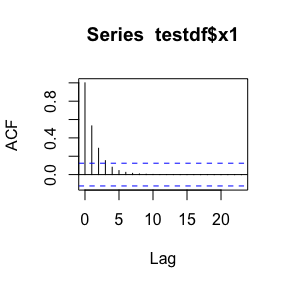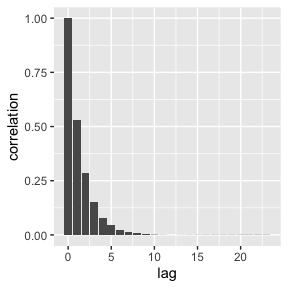qacf(testdf$x1, show_sig = TRUE)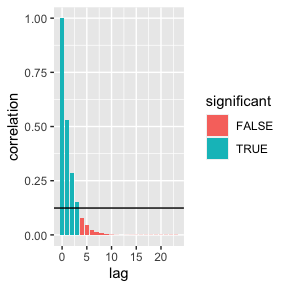# more than one variable acf(testdf)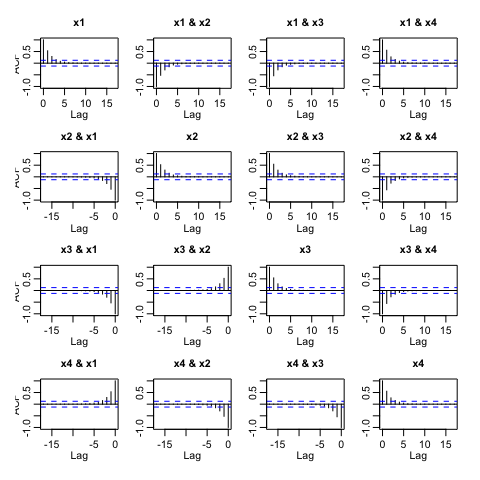qacf(testdf)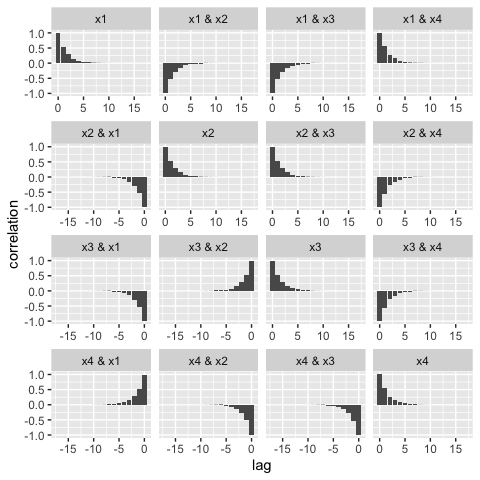qacf(testdf, show_sig = TRUE)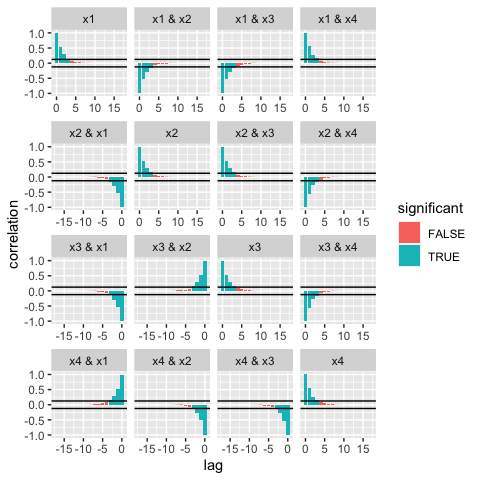## 1.1 Tips and tricks The implementation of qacf is based on the use of stats::acf to produce the statistics needed for the plot. If you want to get at the data itself to build your own acf plot you can extract the data frame from the qacf return: acf_plot_data <- qacf(testdf)$data
##   lag variable      value significant facets
## 1   0       V1 1.00000000        TRUE     x1
## 2   1       V1 0.53067306        TRUE     x1
## 3   2       V1 0.28669463        TRUE     x1
## 4   3       V1 0.15161298        TRUE     x1
## 5   4       V1 0.08015682       FALSE     x1
## 6   5       V1 0.04355315       FALSE     x1

# 2 qblandaltman: Bland Altman Plot

Introduced in [@altman1983measurement] and [@bland1986statistical], the qblandaltman method builds ggplot2 style Bland Altman plots. For examples we use the provided pefr data set which was transcribed from [@bland1986statistical]. See vignette("qwraps2-data-sets", package = "qwraps2") For more details on that data set.

The following replicates the figures in [@bland1986statistical].

Using the first measurement only:

pefr_m1 <-
cbind("Large" = pefr[pefr$measurement == 1 & pefr$meter == "Wright peak flow meter", "pefr"],
"Mini"  = pefr[pefr$measurement == 1 & pefr$meter == "Mini Wright peak flow meter", "pefr"])

A standard x-y style plot and a correlation coefficient suggests that the two meters provide reasonably similar results.

cor(pefr_m1)
##           Large      Mini
## Large 1.0000000 0.9432794
## Mini  0.9432794 1.0000000

ggplot2::ggplot(data = as.data.frame(pefr_m1)) +
ggplot2::aes(x = Large, y = Mini) +
ggplot2::geom_point() +
ggplot2::xlab("Large Meter") +
ggplot2::ylab("Mini Meter") +
ggplot2::xlim(0, 800) +
ggplot2::ylim(0, 800) +
ggplot2::geom_abline(slope = 1)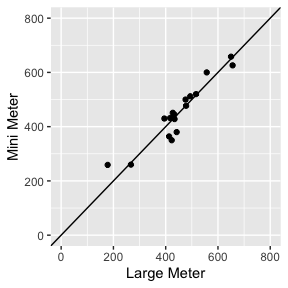However, for many reasons, the above is misleading. One simple note: correlation is not a metric for agreement, i.e., perfect agreement would be shown if all the data points fell on the line of equality whereas perfect correlation occurs when the data points are simply co-linear.

The Bland Altman plot plots the average value on the x-axis and the difference in the measurements on the y-axis:

# default plot
qblandaltman(pefr_m1)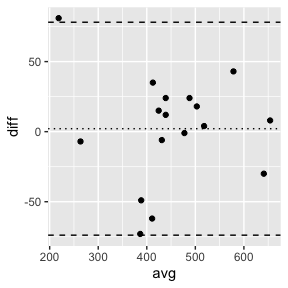# modified plot
ggplot2::last_plot() +
ggplot2::xlim(0, 800) +
ggplot2::ylim(-100, 100) +
ggplot2::xlab("Average of two meters") +
ggplot2::ylab("Difference in the measurements")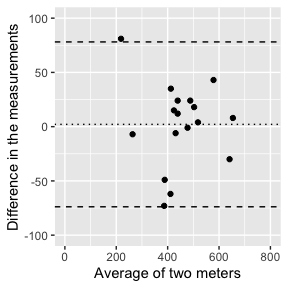There is no distinct relationship between the differences and the average, but the difference in the measurements between the two meters was observed to range between -73 and 81 liters per minute. Such a discrepancy between the meters is not observable from the simple x-y plot.

Reliability, or repeatability, of measurements can also be investigated with a Bland Altman plot.

pefr_mini <-
cbind(m1 = pefr[pefr$measurement == 1 & pefr$meter == "Mini Wright peak flow meter", "pefr"],
m2 = pefr[pefr$measurement == 2 & pefr$meter == "Mini Wright peak flow meter", "pefr"])

qblandaltman(pefr_mini)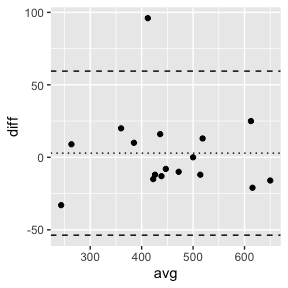# 3 qkmplot: Kaplan Meier Plots

# create a survfit object
require(survival)
leukemia.surv <- survival::survfit(survival::Surv(time, status) ~ x, data = survival::aml)

# base R km plot
survival:::plot.survfit(leukemia.surv, conf.int = TRUE, lty = 2:3, col = 1:2)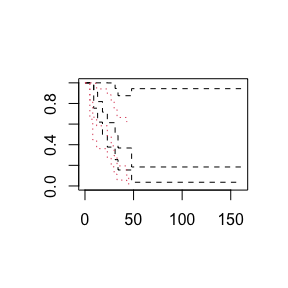# qkmplot
qkmplot(leukemia.surv, conf_int = TRUE)
## Warning: Removed 1 rows containing non-finite values (stat_step_ribbon()).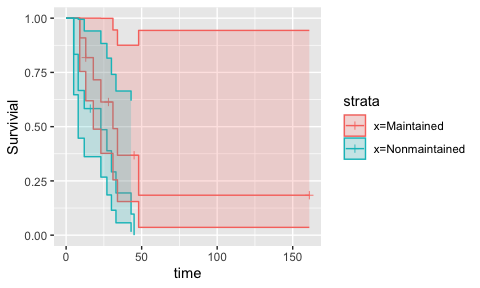The function qkmplot_bulid_data_frame can be used to generate a data.frame needed for building a KM plot. This could be helpful for creating bespoke plots.

leukemia_km_data <- qkmplot_bulid_data_frame(leukemia.surv)
##   time n.risk n.event n.censor      surv upper     lower       strata
## 2    0     11       0        0 1.0000000     1 1.0000000 x=Maintained
## 3    9     11       1        0 0.9090909     1 0.7541338 x=Maintained
## 4   13     10       1        1 0.8181818     1 0.6192490 x=Maintained
qkmplot(leukemia_km_data)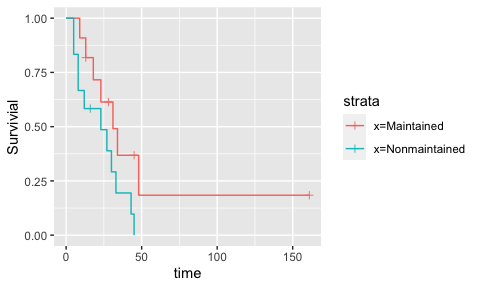Intercept only models are easy to plot too.

intonly_fit <- survival::survfit(survival::Surv(time, status) ~ 1, data = survival::aml)
survival:::plot.survfit(intonly_fit, conf.int = TRUE)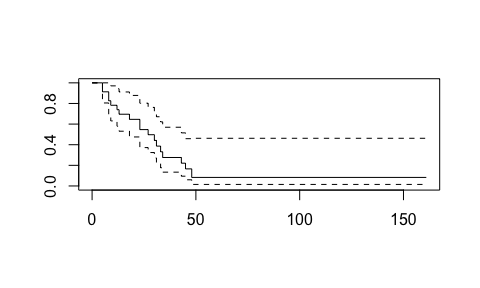qkmplot(intonly_fit, conf_int = TRUE)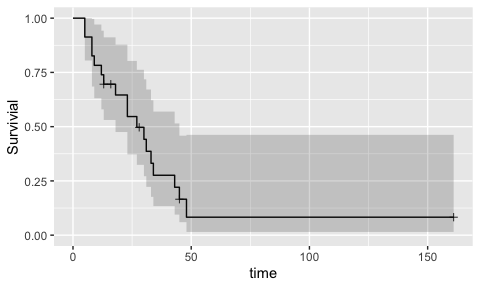# 4 qroc and qprc: Receiver Operating Curve and Precision Recall Curve

Starting in qwraps2 version 0.6.0, the methods for building these graphics have been fundamentally changed as part of a major refactor of the confusion_matrix method which replaces a lot of the code that qroc and qprc were built on.

For this work we will consider a couple models for categorizing email and spam or not based on the Spambase [@spambase] data. More details on this data set can be found in the vignette("qwraps2-data-sets", package = "qwraps2")

Start by defining a training and validation splits of the spambase data

set.seed(42)
tidx <- runif(nrow(spambase)) <= 0.80
xidx <- which(names(spambase) != "spam")
yidx <- which(names(spambase) == "spam")
training_set   <- spambase[tidx, ]
validating_set <- spambase[!tidx, ]

Train a few models:

logistic_model <-
glm(
spam ~ .
, data = training_set
, family = binomial()
)
## Warning: glm.fit: fitted probabilities numerically 0 or 1 occurred

ridge_model <-
glmnet::cv.glmnet(
y = training_set[, yidx]
, x = as.matrix(training_set[, xidx])
, family = binomial()
, alpha = 0
)

lasso_model <-
glmnet::cv.glmnet(
y = training_set[, yidx]
, x = as.matrix(training_set[, xidx])
, family = binomial()
, alpha = 1
)

Generate the predicted values on the validation set:

validating_set$logistic_model_prediction <- predict( logistic_model , newdata = validating_set , type = "response" ) validating_set$ridge_model_prediction <-
as.numeric(
predict(
ridge_model
, newx = as.matrix(validating_set[, xidx])
, type = "response"
, s = "lambda.1se"
)
)

validating_set$lasso_model_prediction <- as.numeric( predict( lasso_model , newx = as.matrix(validating_set[, xidx]) , type = "response" , s = "lambda.1se" ) ) To build ROC and/or PRC plots start by building the confusion matrix for each model. The qwraps2 function confusion_matrix makes this easy. cm1 <- confusion_matrix(spam ~ logistic_model_prediction, data = validating_set) cm2 <- confusion_matrix(spam ~ ridge_model_prediction, data = validating_set) cm3 <- confusion_matrix(spam ~ lasso_model_prediction, data = validating_set) The ROC and PRC plots are ggplot objects and can be modified as you would any other ggplot object. qroc(cm1) + ggplot2::ggtitle("Logisitic Model")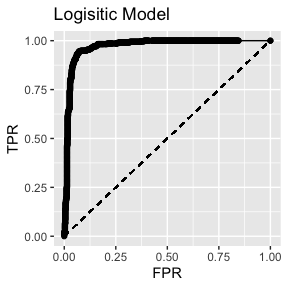qroc(cm2) + ggplot2::ggtitle("Ridge Regression Model")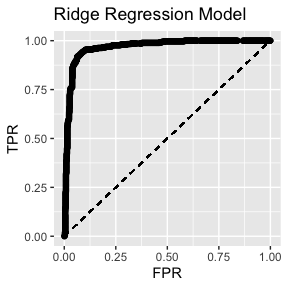qroc(cm3) + ggplot2::ggtitle("LASSO Regression Model")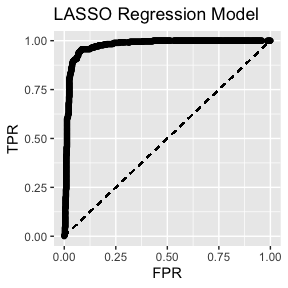Graphing all three curves in one image with AUROC in the legend: roc_plot_data <- rbind( cbind(Model = paste("Logisitic; AUROC =", frmt(cm1$auroc, 3)), cm1$cm_stats) , cbind(Model = paste("Ridge; AUROC =", frmt(cm2$auroc, 3)), cm2$cm_stats) , cbind(Model = paste("LASSO; AUROC =", frmt(cm3$auroc, 3)), cm3$cm_stats) ) qroc(roc_plot_data) + ggplot2::aes(color = Model) + ggplot2::theme(legend.position = "bottom")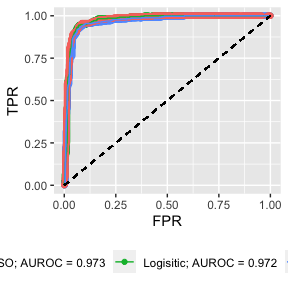Similar for PRC: qprc(cm1) + ggplot2::ggtitle("Logisitic Model")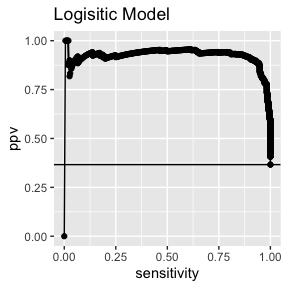qprc(cm2) + ggplot2::ggtitle("Ridge Regression Model")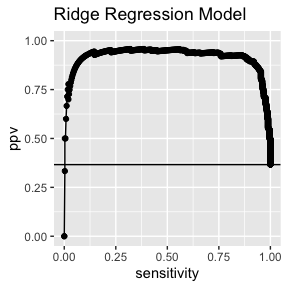qprc(cm3) + ggplot2::ggtitle("LASSO Regression Model")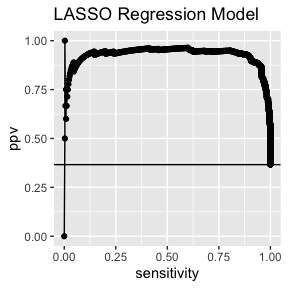prc_plot_data <- rbind( cbind(Model = paste("Logisitic; AUPRC =", frmt(cm1$auprc, 3)), cm1$cm_stats) , cbind(Model = paste("Ridge; AUPRC =", frmt(cm2$auprc, 3)), cm2$cm_stats) , cbind(Model = paste("LASSO; AUPRC =", frmt(cm3$auprc, 3)), cm3$cm_stats) ) qprc(prc_plot_data) + ggplot2::aes(color = Model) + ggplot2::geom_hline(yintercept = cm1$prevalence) +
ggplot2::theme(legend.position = "bottom")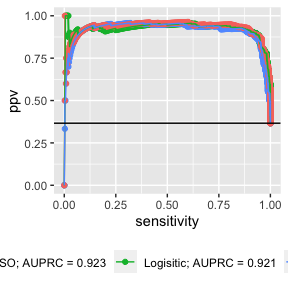# 6 Session Info

sessionInfo()
## R version 4.3.2 (2023-10-31)
## Platform: x86_64-apple-darwin20 (64-bit)
## Running under: macOS Monterey 12.6
##
## Matrix products: default
## BLAS:   /Library/Frameworks/R.framework/Versions/4.3-x86_64/Resources/lib/libRblas.0.dylib
## LAPACK: /Library/Frameworks/R.framework/Versions/4.3-x86_64/Resources/lib/libRlapack.dylib;  LAPACK version 3.11.0
##
## locale:
##  C/en_US.UTF-8/en_US.UTF-8/C/en_US.UTF-8/en_US.UTF-8
##
## time zone: America/Denver
## tzcode source: internal
##
## attached base packages:
##  stats     graphics  grDevices utils     datasets  methods   base
##
## other attached packages:
##  survival_3.5-7 qwraps2_0.6.0
##
## loaded via a namespace (and not attached):
##   glmnet_4.1-8     Matrix_1.6-2     gtable_0.3.4     jsonlite_1.8.7
##   dplyr_1.1.3      compiler_4.3.2   highr_0.10       tidyselect_1.2.0
##   Rcpp_1.0.11      jquerylib_0.1.4  splines_4.3.2    scales_1.2.1
##  yaml_2.3.7       fastmap_1.1.1    lattice_0.22-5   ggplot2_3.4.4
##  R6_2.5.1         labeling_0.4.3   generics_0.1.3   shape_1.4.6
##  knitr_1.45       iterators_1.0.14 tibble_3.2.1     munsell_0.5.0
##  bslib_0.5.1      pillar_1.9.0     rlang_1.1.2      utf8_1.2.4
##  cachem_1.0.8     xfun_0.41        sass_0.4.7       cli_3.6.1
##  withr_2.5.2      magrittr_2.0.3   foreach_1.5.2    digest_0.6.33
##  grid_4.3.2       lifecycle_1.0.4  vctrs_0.6.4      evaluate_0.23
##  glue_1.6.2       farver_2.1.1     codetools_0.2-19 fansi_1.0.5
##  colorspace_2.1-0 rmarkdown_2.25   tools_4.3.2      pkgconfig_2.0.3
##  htmltools_0.5.7